Question of The Day25-02-2022

Select the number-pair in which the two numbers are related in the same way as the two numbers of the following pair:

53 : 34

Correct Answer : c ) 31 : 10

Explanation :

According to the question

The common logic applied here is

Sum of the square of first number is the second number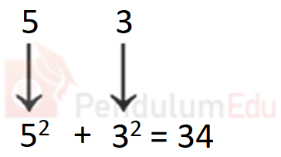Consider option (a)

38 : 70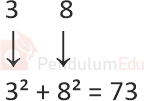73 ≠70

Consider option (b)

24 : 13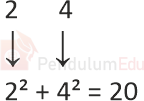20 ≠13

Consider option (c)

31 : 10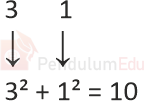10 = 10

Consider option (d)

91: 75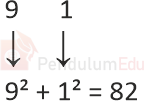82 ≠75

Only option (c) follows the same logic as the number-pair given in the question.

Hence, (c) is the correct answer.0